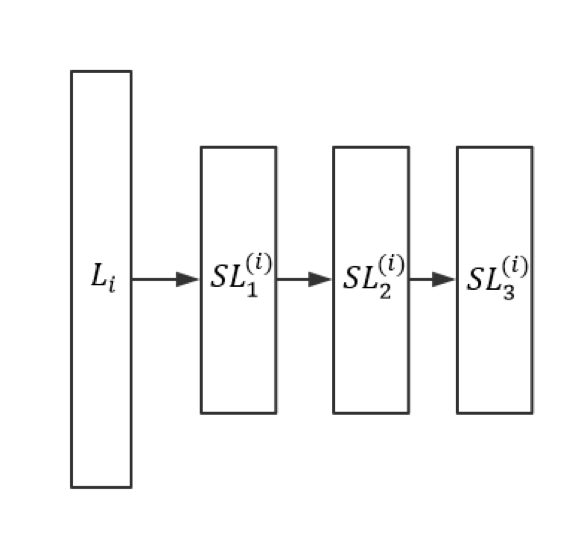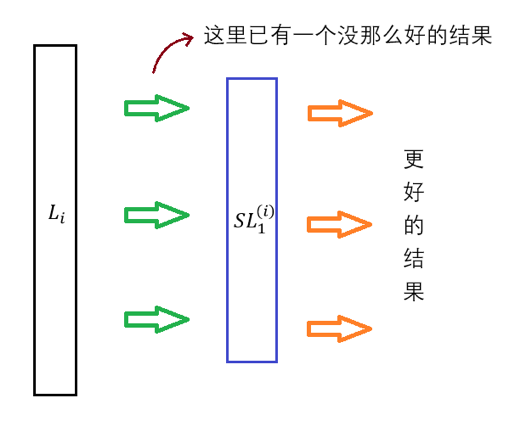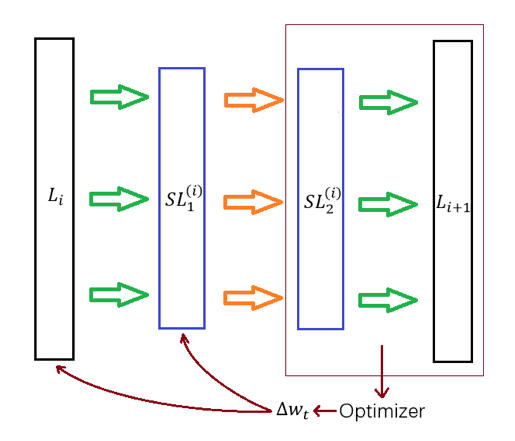CostLayer 算是一个比较特殊的 SubLayer：它附加在输出层的后面、能够根据输出进行相应的变换并得到模型的损失。“根据输出得到损失”即是 CostLayer 实现的特定的功能。对于一般的 SubLayer、它的思想是清晰的：为了在 Layer 的输出的基础上进行一些变换以得到更好的输出；换句话说、SubLayer 通常可以优化 Layer 的输出• $L_{i},SL_{1}^{\left( i \right)},SL_{2}^{\left( i \right)}$分别为$SL_{1}^{\left( i \right)},SL_{2}^{\left( i \right)},SL_{3}^{\left( i \right)}$的父层
• $SL_{1}^{\left( i \right)},SL_{2}^{\left( i \right)},SL_{3}^{\left( i \right)}$分别为$L_{i},SL_{1}^{\left( i \right)},SL_{2}^{\left( i \right)}$的子层
• $L_{i}$$SL_{1}^{\left( i \right)},SL_{2}^{\left( i \right)},SL_{3}^{\left( i \right)}$的 Root Layer
• $SL_{3}^{\left( i \right)}$$L_{i}$的 Leaf Layer

• Layer 和 Layer 之间的关联即为相应的权值矩阵，比如$L_{i},L_{i + 1}$之间的关联即为$w^{\left( i \right)}$
• SubLayer 之间的关联亦即 SubLayer 和 Root Layer 之间的关联都只是“占位符”、它们没有任何实际的作用。这其实是符合 SubLayer 作为“局部优化器”的定位的

• 在前向传导中、它会根据自身的属性和算法来优化从父层处得到的更新
• 在反向传播中、它会有如下三种行为：
• SubLayer 之间的关联以及 SubLayer 和 Root Layer 之间的关联不会被更新、因为它们仅仅是占位符
• SubLayer 作为“局部优化器”、本身可能会有一些参数，这些参数则可能会被 BP 算法更新、但影响域仅在该 SubLayer 的内部（Normalize 会是一个很好的例子）
• Layer 之间的关联的更新是通过 Leaf Layer 完成的。具体而言、$L_{i}$的 Leaf Layer 会利用$L_{i}$的激活函数来完成局部梯度的计算• Dropout 的核心思想在于提高模型的泛化能力：它会在每次迭代中依概率去掉对应 Layer 的某些神经元，从而每次迭代中训练的都是一个小的神经网络
• Normalize 的核心思想在于把父层的输出进行“归一化”、从而期望能够解决由于网络结构过深而引起的“梯度消失”等问题